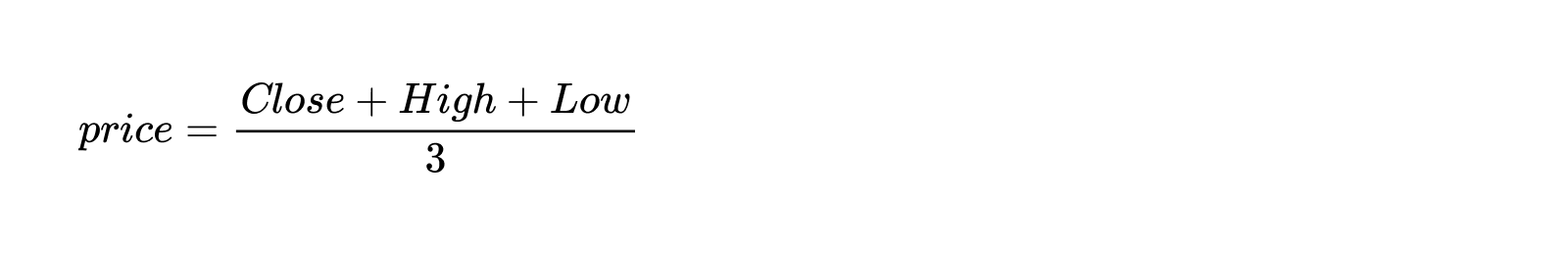# Woodies CCI

#### Definition

The Woodies Commodity Channel Index (CCI) indicator is a modification of the original CCI indicator that is used by traders to help determine turning points. The Woodies CCI indicator consists of two CCIs that are set with the periods 6 and 14, one being a fast curve that is used to track and foresee the movement’s of the slower CCI, the other a slow curve. A histogram is also used to track the results of the slow CCI and is expressed with various colors.

#### History

This Woodie CCI indicator belongs to the oscillators class, it was created by Donald Lambert in the 80s of the last century.

#### Calculations

The following formula is used to calculate the Woodies CCI indicator:def price = close + low + high

def linDev = lindev (price, length)

plot CCI = if linDev == 0 then 0 else (price - Average (price, length)) / linDev / 0.015

plot OverBought = over_bought

plot ZeroLine = 0

plot OverSold = over_sold

Let’s dive a little deeper into these definitions.

Typical price is determined by the average between the high, low, and the close. A division into three is not important in this case because the ratio is calculated at the end.

Linde, or linDev, stands for linear deviations and is determined by the average of the absolute linear deviations of the price from its average over the period = Period. See the formula below, where 0.015 represents a value determined by the script author.#### Takeaways

The Woodies CCI indicator consists of two CCI, one fast and the other slow, in addition to a slow CCI histogram that ranges in color. The colors of the histogram depend on a range of factors and are explained below.

The histogram may consist of any of these four colors: red, green, gray, and yellow. The histogram is gray for 4 bars while a slow CCI crosses the zero line. If the slow CCI is no longer crossing the zero line after this time, the histogram will change to a yellow color in its 5th bar. From the 6th bar and onwards, the histogram will turn red if the CCI is below the zero line, or green if the CCI is above the zero line.

The Woodies CCI indicator has only two parameters: the CCI Period and the TCCI Period.

The CCI Period is the period of a slow CCI.

The TCCI Period is the period of a fast CCI.

You can find recommended values for hours timeframes via our online community and by engaging with experienced traders.

#### What to look for

The Woodies CCI indicators oscillate near the zero line. If the indicator’s value is high, it is highlighting the overbought price. If the indicator’s value is low, it is highlighting the oversold price. Sometimes the indicator’s value is equal to zero. For cases such as these, traders should remember that they themselves ultimately determine the figure in the denominator of the equation for the Woodies CCI and can check their equation to make sure it is not causing any data anomalies.

#### Summary

The Woodies Commodity Channel Index (CCI) indicator helps determine accurate turning points within the financial market. It consists of two CCIs, one a fast curve that is used to track and foresee the movement’s of the slower CCI, and the other slow. Additionally, a histogram is used to track the results of the slow CCI and is expressed with various colors that determine the indicator’s values.